### Spin states, magnetic fields, and electromagnetic radiation

In the presence of an applied external magnetic field, 1H and 13C (also 19F and 31P) nuclei exist in two nuclear spin states of different energy. Slightly more than half of the nuclei exist in the lower energy state called alpha (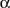) than in the higher energy state called beta (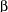). The external magnetic field Bo, which is homogeneous, is created with a large magnet, commonly a super-conducting solenoid. The energy difference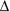E between the spin states is proportional to the strength of Bo. A typical Bo field is 7.05 T (Tesla) or 70,500 Gauss. NMR spectroscopy records transitions between these spin states induced by a radio frequency electromagnetic field called the B1 field. With an applied magnetic field of 7.05 T, the frequency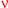of the B1 field is about 300 MHz (megahertz, million cycles per second) for 1H NMR or 75 MHz for 13C NMR. Correspondingly, if the applied field is 11.75 T, the frequency is 500 MHz for 1H NMR or 125 MHz for 13C NMR. Instruments operating at 500 MHz give better sensitivity and resolution than those operating at 300 MHz. The relationship between field and frequency is shown mathematically by Equation 1 and graphically in Figure 1.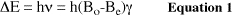where:

•E is the energy difference between spin states
• h is Planck's constant
•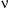is the frequency of the B1 field
• Be is a small magnetic field generated by the circulation of electrons of the molecule (see below)
• Bo is the strength of the external homogeneous magnetic field
•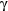(gyromagnetic ratio) is a constant which is a property of the particular nucleus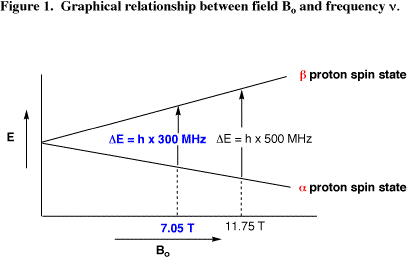Equation 1 introduces the important term Be. In a spherically symmetric s orbital, the Bo field (the external magnetic field) causes the electrons in the cloud surrounding the nucleus to circulate through the orbital (Figure 2). This circulation of electrons produces Be: an induced magnetic field opposed to the Bo field. Thus, the Be field slightly shields the nucleus from the applied magnetic field Bo and the value (Bo–Be) represents the actual magnetic field experienced at the nucleus. The Be field produced by electrons in p and d orbitals are more complex (discussed further in Proton Chemical Shift).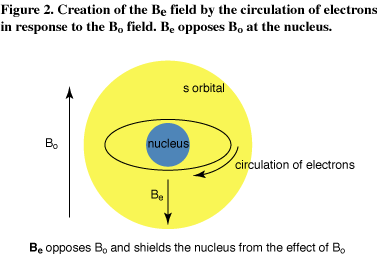The induction of Be is an example of Lenz's Law. The Molecular Expressions web site has an excellent animation of this law.

Figure 2 shows that the Be field slightly shields the nucleus from the applied magnetic field Bo and the value (Bo-Be) represents the actual magnetic field experienced at the nucleus.

If we divide through by h, equation 1 simplifies to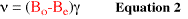or the frequency of a nuclear spin transitionis directly proportional to the magnetic field experienced at the nucleus. This nuclear spin transition frequencyis also called the resonance or precession frequency. The proportionality constantis four times larger for the 1H nucleus than it is for the 13C nucleus. This is why the resonance frequency () is 300 MHz for 1H and only 75 MHz for 13C.

Almost equal population of theandnuclear spin states occurs because the energy difference between the spin states is quite small, 3x10-5 kcal/mol at 300 MHz, and the higher energy state is heavily populated by available thermal energy. The actual population ratio is given by the Boltzmann equation that relates the population ratio toE and the absolute temperature. In contrast, higher energy vibrational states in IR spectroscopy are not significantly populated by available thermal energy because the energy difference between vibrational states is much larger, 5.8 kcal/mol at 2000 cm-1. An excess population of thespin state is required in order to observe an NMR signal, and the slight excess ofspins overspins at 7.05 T is more than sufficient. The excess is higher at higher Bo fields (11.75T/500 MHz versus 7.05 T/300 MHz) becauseE is higher. Correspondingly, a 500 MHz instrument has higher sensitivity than a 300 MHz instrument.

Next section: Chemical ShiftCopyright information: Original content © University of Colorado, Boulder, Chemistry and Biochemistry Department, 2011. The information on these pages is available for academic use without restriction.